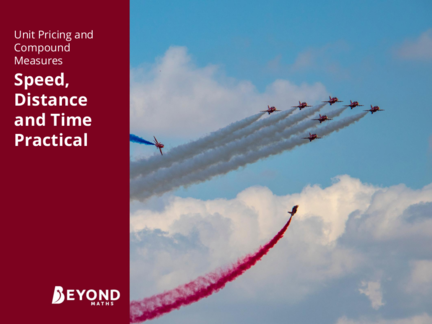# The Speed FormulaContributed by:This pdf includes the following topics:-
Learning Objective
Speed
Equipment
Instructions
Calculating Speed
1. Unit Pricing and
and Time
2. Learning Objective
To collect data and calculate speed.
Success Criteria
• To accurately measure and record time in seconds.
• To accurately measure and record distances in metres.
• To evaluate speed using primary data.
3. Speed
What units do we use to measure speed?
m/s mph km/h
metres per second miles per hour kilometres per hour
How do we measure speed?
How do we calculate speed?
What measurements do we need to calculate speed?
Discuss these questions with your partner.
Step 1 Step 2
Fold the paper in Unfold and then
half to form a fold the corners
crease and then into the centre
unfold the paper. line.
Step 5
Step 3 Step 4
Create a wing
Fold the top Fold the
crease that begins
edges to the plane in half.
at the nose
centre.
5. Equipment
Remember to take a stopwatch, a tape measure,
a pen and your maths book with you.
6. Instructions
1. Locate a start position and put your book on the floor to mark it.
2. One person has the timer ready while the other person stands at the start position
with the paper aeroplane.
3. The person with the timer counts backwards from 3. On ‘1’, start the timer and
throw the paper aeroplane.
4. Stop the timer when the aeroplane lands. Using the tape measure, measure the
distance from the start position to the nose of the aeroplane. Even if the aeroplane
is facing backwards or sideways, you will still need to measure to the nose so the
results remain consistent.
5. Record the time (in seconds) and the distance (in metres) in Flight 1 of the table.
6. Repeat steps 1-5 until every person in the group has flown their paper aeroplane.
7. Once everyone has flown their paper aeroplane, you are ready to start again with
Flight 2.
8. Repeat as many times as you can in the time allocated.
7. Results
Record your flights in the table below. You will need to work with a partner or in a group of three.
Flight Number Time in Seconds Distance in Metres Speed in m/s
1
2
3
4
5
6
7
8
9
10
8. Calculating Speed
To calculate speed, divide the distance travelled by the time taken.
The formula triangle can be used to help
you remember how to calculate speed,
distance or time.
Cover up what you want to find (in this
Distance
case, the speed). The remaining parts
show you how to calculate it.
distance Speed Time
speed =
time
If your aeroplane travelled a distance of 3m and it took 6 seconds for
the flight then the speed is calculated by:
= 0.5m/s
9. Calculating Distance
Use the formula triangle to help.
Cover up what you want to find
Distance (in this case, the distance). The
parts that are left show you how
to calculate it.
Speed Time Distance = Speed × Time
50mph for 3 hours. Calculate the distance.
50 × 3 = 150 miles
10. Calculating Speed
Cover up speed. The parts that are left
show you how to calculate it.
Distance
Speed =
Distance Time
To calculate the average speed of a
journey, you divide the distance
travelled by the time taken.
Speed Time
2 hours to cover a distance of 5.8km. Calculate the speed.
2
= 2.9km/h
11. Calculating Time
Cover up time. The parts that are
left show you how to calculate it.
Distance Time = Distance
Speed
Speed Time
100m travelled at a constant speed of 6.25m/s. Calculate the time taken.
= 16 seconds
12. Your Turn - Section 1
Calculate the distance travelled in each journey:
a. 3 hours at 40mph d. 5.25 hours at 50km/h
Distance = Distance =
Distance = miles Distance = km
b. 55km/h for 3.5 hours e. 30mph for 20 minutes
Distance = Distance =
Distance = km Distance = miles
c. 4 seconds at 3m/s
Distance =
Distance = m
13. Your Turn - Section 1
Calculate the distance travelled in each journey:
a. 3 hours at 40mph d. 5.25 hours at 50km/h
Distance = 3 × 40 Distance = 5.25 × 50
Distance = 120 miles Distance = 262.5km
b. 55km/h for 3.5 hours e. 30mph for 20 minutes
Distance = 55 × 3.5 Distance = 30 × 20
60
Distance = 192.5km Distance = 10 miles
c. 4 seconds at 3m/s
Distance = 4 × 3
Distance = 12m
14. Your Turn - Section 2
Calculate the average speed of each journey:
a. 150 miles in 5 hours d. 87.5 miles in 3.5 hours
Speed = Speed =
Speed = mph Speed = mph
b. 120 km in 3 hours e. 9km in 45 minutes
Speed = Speed =
Speed = km/h Speed = km/h
c. 20m in 4 seconds
Speed =
Speed = m/s
15. Your Turn - Section 2
Calculate the average speed of each journey:
a. 150 miles in 5 hours d. 87.5 miles in 3.5 hours
150 87.5
Speed = Speed =
5 3.5
Speed = 30mph Speed = 25mph
b. 120 km in 3 hours e. 9km in 45 minutes
120 9
Speed = Speed = 9 ÷
3 45
Speed = 40km/h Speed = 12km/h
c. 20m in 4 seconds
20
Speed =
4
Speed = 5m/s
Calculate the speed of each of
distance
speed =
time
17. Summary
improve the speed of your aeroplane.
Consider the following questions.